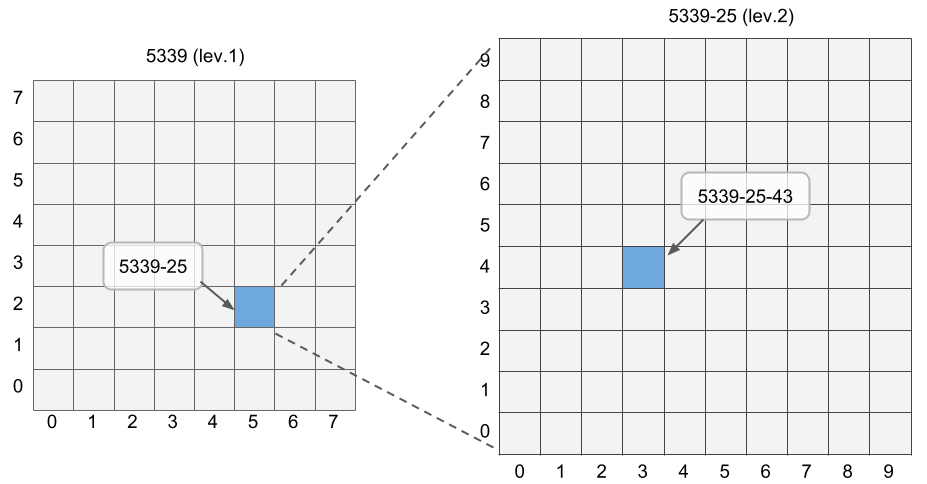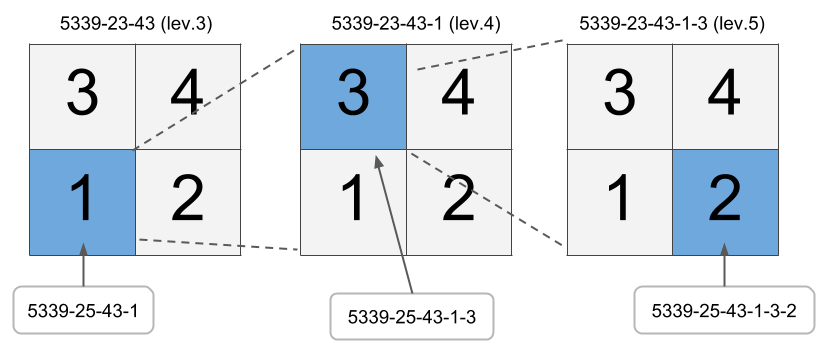# Japanese Mesh GIS Data

## Japanese Mesh GIS Data

This notebook demonstrates:

1. How to read mesh GIS data of Japan.
2. How to visualize mesh areas on an interactive map.
3. How to visualize mesh areas on a static map.
4. How the mesh GIS areas are defined in Japan.

in Python. We will use the following libraries in addition to standard data analysis libraries:

### Data preparation

We use shape files publicly available.

• Shape file of 250m mesh (level 5): Download link. Extract all files in the directory `mesh5/`. If the link does not work, go to the e-stat GIS page and find the 250m mesh data for area `5339`.
• Shape file of 100m mesh: Download link. Extract all files in the directory `land14/`. If the link does not work, go to National Land Numerical Information and download the shape file for area `5339`.

### Libraries

Anaconda/minicoda is recommended.

### Understanding mesh polygon data

The shape file can be downloaded from e-stat. Files are separated by the level 1 mesh. We picked an area `5339` as an example. We will see where it is later.

KEY_CODE MESH1_ID MESH2_ID MESH3_ID MESH4_ID MESH5_ID OBJ_ID geometry
0 5339000011 5339 00 00 1 1 1 POLYGON ((139.003125 35.33333333333333, 139 35...
1 5339000012 5339 00 00 1 2 2 POLYGON ((139.00625 35.33333333333333, 139.003...
2 5339000013 5339 00 00 1 3 3 POLYGON ((139.003125 35.33541666666667, 139 35...
3 5339000014 5339 00 00 1 4 4 POLYGON ((139.00625 35.33541666666667, 139.003...
4 5339000021 5339 00 00 2 1 5 POLYGON ((139.009375 35.33333333333333, 139.00...
KEY_CODE MESH1_ID MESH2_ID MESH3_ID MESH4_ID MESH5_ID OBJ_ID geometry
100795 5339779934 5339 77 99 3 4 100796 POLYGON ((139.99375 35.99791666666666, 139.990...
100796 5339779941 5339 77 99 4 1 100797 POLYGON ((139.996875 35.99583333333333, 139.99...
100797 5339779942 5339 77 99 4 2 100798 POLYGON ((140 35.99583333333333, 139.996875 35...
100798 5339779943 5339 77 99 4 3 100799 POLYGON ((139.996875 35.99791666666666, 139.99...
100799 5339779944 5339 77 99 4 4 100800 POLYGON ((140 35.99791666666666, 139.996875 35...
``````(100800, 8)
``````

Level 5 mesh polygons are supposed to be squares of 250m edges. Let's confirm that. According to this page, 250m is approximately equal to 7.5/3600 latitude and 11.25/3600 = lontitude.

minx miny maxx maxy
100796 139.99375 35.995833 139.996875 35.997917

``````expected longitude (x) edge 0.003125
expected latitude (y) edge 0.0020833333333333333
``````

minx miny maxx maxy
0 139.000000 35.333333 139.003125 35.335417
1 139.003125 35.333333 139.006250 35.335417
2 139.000000 35.335417 139.003125 35.337500
3 139.003125 35.335417 139.006250 35.337500
4 139.006250 35.333333 139.009375 35.335417
``````edge of x axis [0.003125 0.003125]
edge of y axix [0.00208333 0.00208333]
``````

Nice! We got the expected results. Although we got two "unique" numbers, they are almost equal. The mesh shapes are essentially idential.

Next, we will check the edge length for other levels. Edge lengths are supposed to be:

• level 4: 500m
• level 3: 1km
• level 2: 10km
• level 1: 80km

For convenience, we define the functions to convert longitude, latitude to kilometers.

``````0.24999600000000002
0.25
``````

``````level 4 mesh square edges in km
x: [0.5 0.5] y: [0.5 0.5]
level 3 mesh square edges in km
x: [1. 1.] y: [1. 1.]
level 2 mesh square edges in km
x: [10.] y: [10. 10.]
level 1 mesh square edges in km
x: [80.] y: [80.]
``````

The results are exactly as expected!

### Plot mesh on mapTurns out the mesh `5339` covers greater Tokyo area.

Let's further look at the more granular mesh areas.So, this is the area for Bunkyo-ku, Tokyo. Mapping between municipalities and the mesh code (level 3) is found at this page.

### Understanding the rules of mesh code

According to this page, mesh area codes are given by the following rules.

• Each level 1 mesh is split into 8 by 8 grids, making 64 mesh areas of level 2. Rows and columns are counted from 0 to 7, with the south-west corner as origin. Level 2 mesh at the `(x, y)` location is given a code "yx".
• Each level 2 mesh is split into 10 by 10 grids, making 100 mesh areas of level 3. Naming convention is same as above.
• Each level 3 mesh is split into 2 by 2 grids, making 4 mesh areas of level 4. Level 4 mesh areas are named as:
• South-west: 1
• South-east: 2
• North-west: 3
• North-east: 4
• Level 4 and Level 5 are split and named analogously.

Example.Let's see in the real data that the mesh areas are named in this manner.

To do so, we will plot the code as text in the center of mesh cells. Ideally, we would like to show the code on the leaflet map, but that feature has not implemented as of now Link to the issue.

So, we will take another approach of plotting on the map. Library TileMapBase retrieves map data from OpenStreetMap and use it as the background of a plot. Then you can draw on the map as you draw with `matplotlib` library.We can see that all mesh codes are allocated as expected!

### 1/10 or 100m mesh

We sometimes use a more granular mesh definition. One such example is 1/10 mesh, with 100m edges. According to this page, this splits the level 3 mesh into 10 by 10 grids. However, the page does not clearly state how the mesh codes are defined. Let's take a look.

We use an example of land use data obtained from here.

メッシュ 土地利用種 geometry
0 5339000000 0500 POLYGON ((139 35.33333333333334, 139 35.334166...
1 5339000001 0500 POLYGON ((139.00125 35.33333333333334, 139.001...
2 5339000002 0500 POLYGON ((139.0025 35.33333333333334, 139.0025...
3 5339000003 0500 POLYGON ((139.00375 35.33333333333334, 139.003...
4 5339000004 0500 POLYGON ((139.005 35.33333333333334, 139.005 3...
メッシュ 土地利用種 geometry
458968 5339779995 0703 POLYGON ((139.99375 35.99916666666667, 139.993...
458969 5339779996 0100 POLYGON ((139.995 35.99916666666667, 139.995 3...
458970 5339779997 0100 POLYGON ((139.99625 35.99916666666667, 139.996...
458971 5339779998 0100 POLYGON ((139.9975 35.99916666666667, 139.9975...
458972 5339779999 0100 POLYGON ((139.99875 35.99916666666667, 139.998...
``````(458973, 3)
``````

``````edge x: [0.1]
edge y: [0.1]
``````

Mesh areas area square with edge of 100m.We can see that the naming rule is same as the level 3 mesh; Rows and columns are indexed from zero, with the origin at the south-west. Then, cell at `(x, y)` position is named as "yx". We can now see how we should match 100m mesh to level 4 mesh (500m):

• `y <= 4, x <= 4` --> Mesh4 code = 1
• `y <= 4, x >= 5` --> Mesh4 code = 2
• `y >= 5, x <= 4` --> Mesh4 code = 3
• `y >= 5, x >= 5` --> Mesh4 code = 4